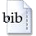User: GuestLogin
Original title:
Über die Tiefe von Invariantenringen unendlicher Gruppen
Translated title:
On the depth of invariant rings of infinite groups
Author:
Kohls, MartinYear:
2007
Document type:
Dissertation
Faculty/School:
Fakultät für Mathematik
Kemper, Gregor (Prof. Dr.)
Referee:
Derksen, Harm (Prof. Dr.)
Language:
de
Subject group:
MAT Mathematik
Keywords:
Invariantentheorie, Cohen-Macaulay, Tiefe, Kohomologie, algebraische Gruppe, Vektorinvarianten, linear reduktiv
Translated keywords:
invariant theory, Cohen-Macaulay, depth, cohomology, algebraic group, vector invariants, linearly reductive
Controlled terms:
Lineare algebraische Gruppe; Kohomologietheorie; Invariantentheorie; Cohen-Macaulay-Ring; Cohen-Macaulay-Modul
TUM classification:
MAT 120d; MAT 130d; MAT 143d
Abstract:
Sei K ein algebraisch abgeschlossener Körper, G eine über K definierte lineare algebraische Gruppe und V ein G-Modul. Wir geben obere Schranken für die Tiefe depth K[V]^G bzw. äquivalent hierzu untere Schranken für den Cohen-Macaulay-Defekt cmdef K[V]^G=dim K[V]^G-depth K[V]^G. Solche Schranken existieren bisher nur für endliches G. Insbesondere zeigen wir folgendes Resultat: Für jede reduktive, nicht linear reduktive Gruppe G existiert ein treuer G-Modul V (den wir explizit angeben) mit cmdef K...     »
Translated abstract:
Let K be an algebraically closed field, G a linear algebraic group defined over K and V a G-module. We give upper bounds for the depth of K[V]^G, or equivalently lower bounds for the Cohen-Macaulay-Defect cmdef K[V]^G=dim K[V]^G-depth K[V]^G. So far, such bounds are only known when G is finite. In particular, we show the following result: For each reductive, but not linearly reductive group G, there exists a faithful G-module V (which we give explicitely) such that cmdef K[sum_{i=1}^{n} V]^G >=...     »
WWW:
https://mediatum.ub.tum.de/?id=619331
Date of submission:
15.05.2007
Oral examination:
12.11.2007
Pages:
152
Urn (citeable URL):
https://nbn-resolving.de/urn/resolver.pl?urn:nbn:de:bvb:91-diss-20071116-619331-1-3
Last change:
24.09.2008BibTeX